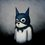# Josephus Problem

The First Algorithm is the solution to the Josephus Problem.

The problem is based on a tale during the Roman Empire, 41 jews were hiding in a cave and were about to be captured by the Romans but the jews preferred suicide over surrender and hence devised a strategy. They all would stand in a circle and every third person would be killed. Josephus did not want to commit suicide and as he was a smart man he calculated the exact position where he should stand so he is the last man standing.

The Modern problem is based on a similar lines but instead of 41, n people are given and instead of every third person to be killed every k person is killed. You have to find the safe position

Say n = 5 and k = 2, then the order of killing is 2,4,1,5

Here we observe that after the first kill i.e the killing of second person we have n-1 people and so the problem can be considered as finding the safe position with n-1 people when person on k position is executed. So, the problem has a nice recursive structure but the only problem is after the first kill the k+1 position person assumes the first position which can be adjusted as $k \% n + 1$

Algorithm:

 1 2 3 4 5 function josephus(n, k): if n == 1: return n else: return (josephus(n-1,k) + k) % n + 1 

For a nice visualisation of the Josephus Problem visit the following link: JosephusNote by Gaurav Pathak
7 years, 1 month ago

This discussion board is a place to discuss our Daily Challenges and the math and science related to those challenges. Explanations are more than just a solution — they should explain the steps and thinking strategies that you used to obtain the solution. Comments should further the discussion of math and science.

When posting on Brilliant:

• Use the emojis to react to an explanation, whether you're congratulating a job well done , or just really confused .
• Ask specific questions about the challenge or the steps in somebody's explanation. Well-posed questions can add a lot to the discussion, but posting "I don't understand!" doesn't help anyone.
• Try to contribute something new to the discussion, whether it is an extension, generalization or other idea related to the challenge.

MarkdownAppears as
*italics* or _italics_ italics
**bold** or __bold__ bold
- bulleted- list
• bulleted
• list
1. numbered2. list
1. numbered
2. list
Note: you must add a full line of space before and after lists for them to show up correctly
paragraph 1paragraph 2

paragraph 1

paragraph 2

[example link](https://brilliant.org)example link
> This is a quote
This is a quote
    # I indented these lines
# 4 spaces, and now they show
# up as a code block.

print "hello world"
# I indented these lines
# 4 spaces, and now they show
# up as a code block.

print "hello world"
MathAppears as
Remember to wrap math in $$ ... $$ or $ ... $ to ensure proper formatting.
2 \times 3 $2 \times 3$
2^{34} $2^{34}$
a_{i-1} $a_{i-1}$
\frac{2}{3} $\frac{2}{3}$
\sqrt{2} $\sqrt{2}$
\sum_{i=1}^3 $\sum_{i=1}^3$
\sin \theta $\sin \theta$
\boxed{123} $\boxed{123}$